16 Waves

16.1 Traveling Waves

Learning Objectives

By the end of this section, you will be able to:

• Describe the basic characteristics of wave motion
• Define the terms wavelength, amplitude, period, frequency, and wave speed
• Explain the difference between longitudinal and transverse waves, and give examples of each type
• List the different types of waves

We saw in Oscillations that oscillatory motion is an important type of behavior that can be used to model a wide range of physical phenomena. Oscillatory motion is also important because oscillations can generate waves, which are of fundamental importance in physics. Many of the terms and equations we studied in the chapter on oscillations apply equally well to wave motion ((Figure)).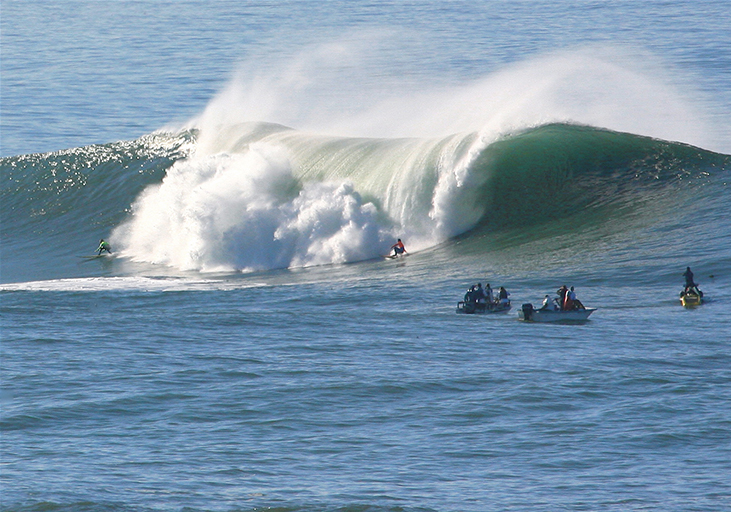Figure 16.2 From the world of renewable energy sources comes the electric power-generating buoy. Although there are many versions, this one converts the up-and-down motion, as well as side-to-side motion, of the buoy into rotational motion in order to turn an electric generator, which stores the energy in batteries.
An ocean wave is probably the first picture that comes to mind when you hear the word “wave.” Although this breaking wave, and ocean waves in general, have apparent similarities to the basic wave characteristics we will discuss, the mechanisms driving ocean waves are highly complex and beyond the scope of this chapter. It may seem natural, and even advantageous, to apply the concepts in this chapter to ocean waves, but ocean waves are nonlinear, and the simple models presented in this chapter do not fully explain them. (credit: Steve Jurvetson)

Types of Waves

A wave is a disturbance that propagates, or moves from the place it was created. There are three basic types of waves: mechanical waves, electromagnetic waves, and matter waves.

Basic mechanical waves are governed by Newton’s laws and require a medium. A medium is the substance a mechanical waves propagates through, and the medium produces an elastic restoring force when it is deformed. Mechanical waves transfer energy and momentum, without transferring mass. Some examples of mechanical waves are water waves, sound waves, and seismic waves. The medium for water waves is water; for sound waves, the medium is usually air. (Sound waves can travel in other media as well; we will look at that in more detail in Sound.) For surface water waves, the disturbance occurs on the surface of the water, perhaps created by a rock thrown into a pond or by a swimmer splashing the surface repeatedly. For sound waves, the disturbance is a change in air pressure, perhaps created by the oscillating cone inside a speaker or a vibrating tuning fork. In both cases, the disturbance is the oscillation of the molecules of the fluid. In mechanical waves, energy and momentum transfer with the motion of the wave, whereas the mass oscillates around an equilibrium point. (We discuss this in Energy and Power of a Wave.) Earthquakes generate seismic waves from several types of disturbances, including the disturbance of Earth’s surface and pressure disturbances under the surface. Seismic waves travel through the solids and liquids that form Earth. In this chapter, we focus on mechanical waves.

Electromagnetic waves are associated with oscillations in electric and magnetic fields and do not require a medium. Examples include gamma rays, X-rays, ultraviolet waves, visible light, infrared waves, microwaves, and radio waves. Electromagnetic waves can travel through a vacuum at the speed of light,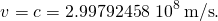For example, light from distant stars travels through the vacuum of space and reaches Earth. Electromagnetic waves have some characteristics that are similar to mechanical waves; they are covered in more detail in Electromagnetic Waves in volume 2 of this text.

Matter waves are a central part of the branch of physics known as quantum mechanics. These waves are associated with protons, electrons, neutrons, and other fundamental particles found in nature. The theory that all types of matter have wave-like properties was first proposed by Louis de Broglie in 1924. Matter waves are discussed in Photons and Matter Waves in the third volume of this text.

Mechanical Waves

Mechanical waves exhibit characteristics common to all waves, such as amplitude, wavelength, period, frequency, and energy. All wave characteristics can be described by a small set of underlying principles.

The simplest mechanical waves repeat themselves for several cycles and are associated with simple harmonic motion. These simple harmonic waves can be modeled using some combination of sine and cosine functions. For example, consider the simplified surface water wave that moves across the surface of water as illustrated in (Figure). Unlike complex ocean waves, in surface water waves, the medium, in this case water, moves vertically, oscillating up and down, whereas the disturbance of the wave moves horizontally through the medium. In (Figure), the waves causes a seagull to move up and down in simple harmonic motion as the wave crests and troughs (peaks and valleys) pass under the bird. The crest is the highest point of the wave, and the trough is the lowest part of the wave. The time for one complete oscillation of the up-and-down motion is the wave’s period T. The wave’s frequency is the number of waves that pass through a point per unit time and is equal to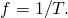The period can be expressed using any convenient unit of time but is usually measured in seconds; frequency is usually measured in hertz (Hz), where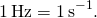The length of the wave is called the wavelength and is represented by the Greek letter lambda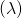, which is measured in any convenient unit of length, such as a centimeter or meter. The wavelength can be measured between any two similar points along the medium that have the same height and the same slope. In (Figure), the wavelength is shown measured between two crests. As stated above, the period of the wave is equal to the time for one oscillation, but it is also equal to the time for one wavelength to pass through a point along the wave’s path.

The amplitude of the wave (A) is a measure of the maximum displacement of the medium from its equilibrium position. In the figure, the equilibrium position is indicated by the dotted line, which is the height of the water if there were no waves moving through it. In this case, the wave is symmetrical, the crest of the wave is a distance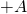above the equilibrium position, and the trough is a distance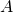below the equilibrium position. The units for the amplitude can be centimeters or meters, or any convenient unit of distance.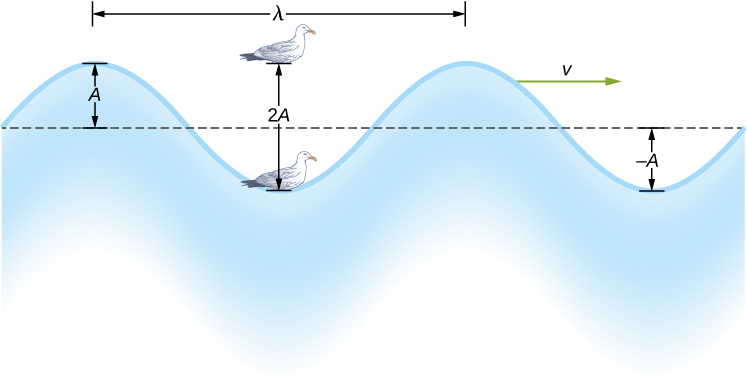Figure 16.3 An idealized surface water wave passes under a seagull that bobs up and down in simple harmonic motion. The wave has a wavelength     , which is the distance between adjacent identical parts of the wave. The amplitude A of the wave is the maximum displacement of the wave from the equilibrium position, which is indicated by the dotted line. In this example, the medium moves up and down, whereas the disturbance of the surface propagates parallel to the surface at a speed v.

The water wave in the figure moves through the medium with a propagation velocity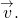The magnitude of the wave velocity is the distance the wave travels in a given time, which is one wavelength in the time of one period, and the wave speed is the magnitude of wave velocity. In equation form, this is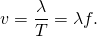This fundamental relationship holds for all types of waves. For water waves, v is the speed of a surface wave; for sound, v is the speed of sound; and for visible light, v is the speed of light.

Transverse and Longitudinal Waves

We have seen that a simple mechanical wave consists of a periodic disturbance that propagates from one place to another through a medium. In (Figure)(a), the wave propagates in the horizontal direction, whereas the medium is disturbed in the vertical direction. Such a wave is called a transverse wave. In a transverse wave, the wave may propagate in any direction, but the disturbance of the medium is perpendicular to the direction of propagation. In contrast, in a longitudinal wave or compressional wave, the disturbance is parallel to the direction of propagation. (Figure)(b) shows an example of a longitudinal wave. The size of the disturbance is its amplitude A and is completely independent of the speed of propagation v.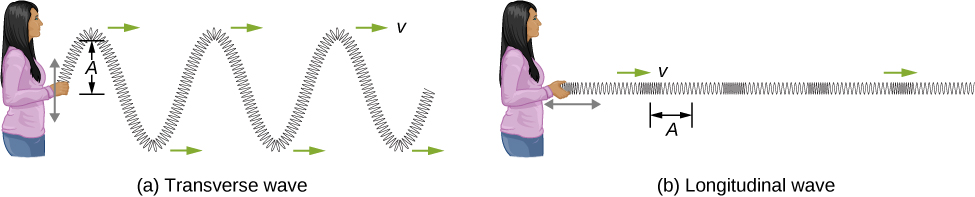Figure 16.4 (a) In a transverse wave, the medium oscillates perpendicular to the wave velocity. Here, the spring moves vertically up and down, while the wave propagates horizontally to the right. (b) In a longitudinal wave, the medium oscillates parallel to the propagation of the wave. In this case, the spring oscillates back and forth, while the wave propagates to the right.

A simple graphical representation of a section of the spring shown in (Figure)(b) is shown in (Figure). (Figure)(a) shows the equilibrium position of the spring before any waves move down it. A point on the spring is marked with a blue dot. (Figure)(b) through (g) show snapshots of the spring taken one-quarter of a period apart, sometime after the end of` the spring is oscillated back and forth in the x-direction at a constant frequency. The disturbance of the wave is seen as the compressions and the expansions of the spring. Note that the blue dot oscillates around its equilibrium position a distance A, as the longitudinal wave moves in the positive x-direction with a constant speed. The distance A is the amplitude of the wave. The y-position of the dot does not change as the wave moves through the spring. The wavelength of the wave is measured in part (d). The wavelength depends on the speed of the wave and the frequency of the driving force.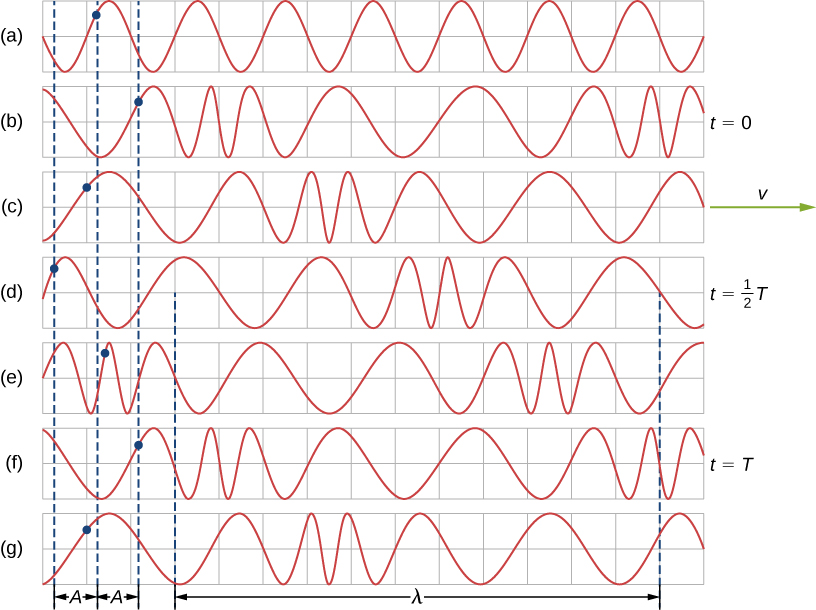Figure 16.5 (a) This is a simple, graphical representation of a section of the stretched spring shown in (Figure)(b), representing the spring’s equilibrium position before any waves are induced on the spring. A point on the spring is marked by a blue dot. (b–g) Longitudinal waves are created by oscillating the end of the spring (not shown) back and forth along the x-axis. The longitudinal wave, with a wavelength     , moves along the spring in the +x-direction with a wave speed v. For convenience, the wavelength is measured in (d). Note that the point on the spring that was marked with the blue dot moves back and forth a distance A from the equilibrium position, oscillating around the equilibrium position of the point.

Waves may be transverse, longitudinal, or a combination of the two. Examples of transverse waves are the waves on stringed instruments or surface waves on water, such as ripples moving on a pond. Sound waves in air and water are longitudinal. With sound waves, the disturbances are periodic variations in pressure that are transmitted in fluids. Fluids do not have appreciable shear strength, and for this reason, the sound waves in them are longitudinal waves. Sound in solids can have both longitudinal and transverse components, such as those in a seismic wave. Earthquakes generate seismic waves under Earth’s surface with both longitudinal and transverse components (called compressional or P-waves and shear or S-waves, respectively). The components of seismic waves have important individual characteristics—they propagate at different speeds, for example. Earthquakes also have surface waves that are similar to surface waves on water. Ocean waves also have both transverse and longitudinal components.

Example

Wave on a String

A student takes a 30.00-m-long string and attaches one end to the wall in the physics lab. The student then holds the free end of the rope, keeping the tension constant in the rope. The student then begins to send waves down the string by moving the end of the string up and down with a frequency of 2.00 Hz. The maximum displacement of the end of the string is 20.00 cm. The first wave hits the lab wall 6.00 s after it was created. (a) What is the speed of the wave? (b) What is the period of the wave? (c) What is the wavelength of the wave?

Strategy

1. The speed of the wave can be derived by dividing the distance traveled by the time.
2. The period of the wave is the inverse of the frequency of the driving force.
3. The wavelength can be found from the speed and the period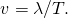Solution

1. The first wave traveled 30.00 m in 6.00 s: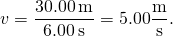2. The period is equal to the inverse of the frequency: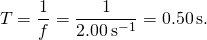3. The wavelength is equal to the velocity times the period: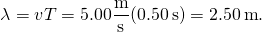Significance

The frequency of the wave produced by an oscillating driving force is equal to the frequency of the driving force.

When a guitar string is plucked, the guitar string oscillates as a result of waves moving through the string. The vibrations of the string cause the air molecules to oscillate, forming sound waves. The frequency of the sound waves is equal to the frequency of the vibrating string. Is the wavelength of the sound wave always equal to the wavelength of the waves on the string?

The wavelength of the waves depends on the frequency and the velocity of the wave. The frequency of the sound wave is equal to the frequency of the wave on the string. The wavelengths of the sound waves and the waves on the string are equal only if the velocities of the waves are the same, which is not always the case. If the speed of the sound wave is different from the speed of the wave on the string, the wavelengths are different. This velocity of sound waves will be discussed in Sound.

Example

Characteristics of a Wave

A transverse mechanical wave propagates in the positive x-direction through a spring (as shown in (Figure)(a)) with a constant wave speed, and the medium oscillates betweenandaround an equilibrium position. The graph in (Figure) shows the height of the spring (y) versus the position (x), where the x-axis points in the direction of propagation. The figure shows the height of the spring versus the x-position at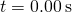as a dotted line and the wave at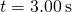as a solid line. (a) Determine the wavelength and amplitude of the wave. (b) Find the propagation velocity of the wave. (c) Calculate the period and frequency of the wave.

Strategy

1. The amplitude and wavelength can be determined from the graph.
2. Since the velocity is constant, the velocity of the wave can be found by dividing the distance traveled by the wave by the time it took the wave to travel the distance.
3. The period can be found from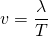and the frequency from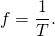Solution

1. Read the wavelength from the graph, looking at the purple arrow in (Figure). Read the amplitude by looking at the green arrow. The wavelength is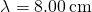and the amplitude is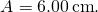2. The distance the wave traveled from timeto timecan be seen in the graph. Consider the red arrow, which shows the distance the crest has moved in 3 s. The distance is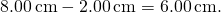The velocity is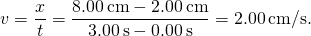3. The period is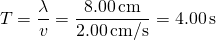and the frequency is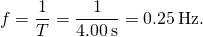Significance

Note that the wavelength can be found using any two successive identical points that repeat, having the same height and slope. You should choose two points that are most convenient. The displacement can also be found using any convenient point.

The propagation velocity of a transverse or longitudinal mechanical wave may be constant as the wave disturbance moves through the medium. Consider a transverse mechanical wave: Is the velocity of the medium also constant?

[hidden-answer a=”148831″]In a transverse wave, the wave may move at a constant propagation velocity through the medium, but the medium oscillates perpendicular to the motion of the wave. If the wave moves in the positive x-direction, the medium oscillates up and down in the y-direction. The velocity of the medium is therefore not constant, but the medium’s velocity and acceleration are similar to that of the simple harmonic motion of a mass on a spring.[/hidden-answer]

Summary

• A wave is a disturbance that moves from the point of origin with a wave velocity v.
• A wave has a wavelength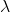, which is the distance between adjacent identical parts of the wave. Wave velocity and wavelength are related to the wave’s frequency and period by• Mechanical waves are disturbances that move through a medium and are governed by Newton’s laws.
• Electromagnetic waves are disturbances in the electric and magnetic fields, and do not require a medium.
• Matter waves are a central part of quantum mechanics and are associated with protons, electrons, neutrons, and other fundamental particles found in nature.
• A transverse wave has a disturbance perpendicular to the wave’s direction of propagation, whereas a longitudinal wave has a disturbance parallel to its direction of propagation.

Conceptual Questions

Give one example of a transverse wave and one example of a longitudinal wave, being careful to note the relative directions of the disturbance and wave propagation in each.

[hidden-answer a=”548814″]A wave on a guitar string is an example of a transverse wave. The disturbance of the string moves perpendicular to the propagation of the wave. The sound produced by the string is a longitudinal wave where the disturbance of the air moves parallel to the propagation of the wave.[/hidden-answer]

A sinusoidal transverse wave has a wavelength of 2.80 m. It takes 0.10 s for a portion of the string at a position x to move from a maximum position of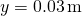to the equilibrium position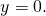What are the period, frequency, and wave speed of the wave?

What is the difference between propagation speed and the frequency of a mechanical wave? Does one or both affect wavelength? If so, how?

Propagation speed is the speed of the wave propagating through the medium. If the wave speed is constant, the speed can be found byThe frequency is the number of wave that pass a point per unit time. The wavelength is directly proportional to the wave speed and inversely proportional to the frequency.

Consider a stretched spring, such as a slinky. The stretched spring can support longitudinal waves and transverse waves. How can you produce transverse waves on the spring? How can you produce longitudinal waves on the spring?

Consider a wave produced on a stretched spring by holding one end and shaking it up and down. Does the wavelength depend on the distance you move your hand up and down?

No, the distance you move your hand up and down will determine the amplitude of the wave. The wavelength will depend on the frequency you move your hand up and down, and the speed of the wave through the spring.

A sinusoidal, transverse wave is produced on a stretched spring, having a period T. Each section of the spring moves perpendicular to the direction of propagation of the wave, in simple harmonic motion with an amplitude A. Does each section oscillate with the same period as the wave or a different period? If the amplitude of the transverse wave were doubled but the period stays the same, would your answer be the same?

An electromagnetic wave, such as light, does not require a medium. Can you think of an example that would support this claim?

[hidden-answer a=”51285″]Light from the Sun and stars reach Earth through empty space where there is no medium present.[/hidden-answer]

Problems

Storms in the South Pacific can create waves that travel all the way to the California coast, 12,000 km away. How long does it take them to travel this distance if they travel at 15.0 m/s?

Waves on a swimming pool propagate at 0.75 m/s. You splash the water at one end of the pool and observe the wave go to the opposite end, reflect, and return in 30.00 s. How far away is the other end of the pool?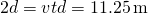Wind gusts create ripples on the ocean that have a wavelength of 5.00 cm and propagate at 2.00 m/s. What is their frequency?

How many times a minute does a boat bob up and down on ocean waves that have a wavelength of 40.0 m and a propagation speed of 5.00 m/s?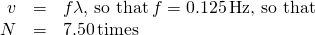Scouts at a camp shake the rope bridge they have just crossed and observe the wave crests to be 8.00 m apart. If they shake the bridge twice per second, what is the propagation speed of the waves?

What is the wavelength of the waves you create in a swimming pool if you splash your hand at a rate of 2.00 Hz and the waves propagate at a wave speed of 0.800 m/s?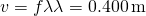What is the wavelength of an earthquake that shakes you with a frequency of 10.0 Hz and gets to another city 84.0 km away in 12.0 s?

Radio waves transmitted through empty space at the speed of light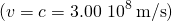by the Voyager spacecraft have a wavelength of 0.120 m. What is their frequency?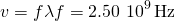Your ear is capable of differentiating sounds that arrive at each ear just 0.34 ms apart, which is useful in determining where low frequency sound is originating from. (a) Suppose a low-frequency sound source is placed to the right of a person, whose ears are approximately 18 cm apart, and the speed of sound generated is 340 m/s. How long is the interval between when the sound arrives at the right ear and the sound arrives at the left ear? (b) Assume the same person was scuba diving and a low-frequency sound source was to the right of the scuba diver. How long is the interval between when the sound arrives at the right ear and the sound arrives at the left ear, if the speed of sound in water is 1500 m/s? (c) What is significant about the time interval of the two situations?

(a) Seismographs measure the arrival times of earthquakes with a precision of 0.100 s. To get the distance to the epicenter of the quake, geologists compare the arrival times of S- and P-waves, which travel at different speeds. If S- and P-waves travel at 4.00 and 7.20 km/s, respectively, in the region considered, how precisely can the distance to the source of the earthquake be determined? (b) Seismic waves from underground detonations of nuclear bombs can be used to locate the test site and detect violations of test bans. Discuss whether your answer to (a) implies a serious limit to such detection. (Note also that the uncertainty is greater if there is an uncertainty in the propagation speeds of the S- and P-waves.)

a. The P-waves outrun the S-waves by a speed of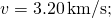therefore,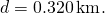b. Since the uncertainty in the distance is less than a kilometer, our answer to part (a) does not seem to limit the detection of nuclear bomb detonations. However, if the velocities are uncertain, then the uncertainty in the distance would increase and could then make it difficult to identify the source of the seismic waves.

A Girl Scout is taking a 10.00-km hike to earn a merit badge. While on the hike, she sees a cliff some distance away. She wishes to estimate the time required to walk to the cliff. She knows that the speed of sound is approximately 343 meters per second. She yells and finds that the echo returns after approximately 2.00 seconds. If she can hike 1.00 km in 10 minutes, how long would it take her to reach the cliff?

A quality assurance engineer at a frying pan company is asked to qualify a new line of nonstick-coated frying pans. The coating needs to be 1.00 mm thick. One method to test the thickness is for the engineer to pick a percentage of the pans manufactured, strip off the coating, and measure the thickness using a micrometer. This method is a destructive testing method. Instead, the engineer decides that every frying pan will be tested using a nondestructive method. An ultrasonic transducer is used that produces sound waves with a frequency of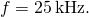The sound waves are sent through the coating and are reflected by the interface between the coating and the metal pan, and the time is recorded. The wavelength of the ultrasonic waves in the coating is 0.076 m. What should be the time recorded if the coating is the correct thickness (1.00 mm)?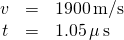Glossary

longitudinal wave
wave in which the disturbance is parallel to the direction of propagation
mechanical wave
wave that is governed by Newton’s laws and requires a medium
transverse wave
wave in which the disturbance is perpendicular to the direction of propagation
wave
disturbance that moves from its source and carries energy
wave velocity
velocity at which the disturbance moves; also called the propagation velocity
wave speed
magnitude of the wave velocity
wavelength
distance between adjacent identical parts of a wave